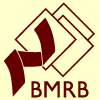# NMR Restraints Grid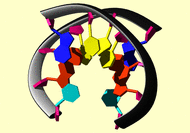Result table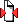(Save to zip file containing files for each block)

 image mrblock_id pdb_id cing stage position program type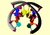476132 1buf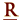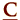cing 1-original 4 AMBER angle

```# assume 'with parabolic regions continuing on either side for 20 degree'
# means r1=r2-20, r4=r3+20, rk2, rk3 = 50 % of kdist (10) = 5
# H-bond angle C1-G12
&rst iat=17,18,365,-1,iresid=0,r1=150,r2=170,r3=190,r4=210,
rk2=5,rk3=5,&end
&rst iat=20,367,366,-1,r2=170,r3=190,&end
&rst iat=22,370,369,-1,r2=170,r3=190,&end
# H-bond angle A2-T11
&rst iat=47,48,336,-1,r2=170,r3=190,&end
&rst iat=50,338,337,-1,r2=170,r3=190,&end
# H-bond angle A3-T10
&rst iat=79,80,304,-1,r2=170,r3=190,&end
&rst iat=82,306,305,-1,r2=170,r3=190,&end
# H-bond angle T4-A9
&rst iat=114,270,269,-1,r2=170,r3=190,&end
&rst iat=115,116,272,-1,r2=170,r3=190,&end
# H-bond angle T5-A8
&rst iat=146,238,237,-1,r2=170,r3=190,&end
&rst iat=147,148,240,-1,r2=170,r3=190,&end
# H-bond angle G6-C7
&rst iat=175,208,207,-1,r2=170,r3=190,&end
&rst iat=176,177,210,-1,r2=170,r3=190,&end
&rst iat=179,180,212,-1,r2=170,r3=190,&end

```

Please acknowledge these references in publications where the data from this site have been utilized.

Contact the webmaster for help, if required. Wednesday, October 20, 2021 11:42:57 PM GMT (wattos1)